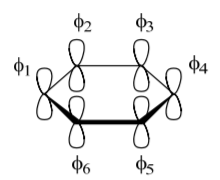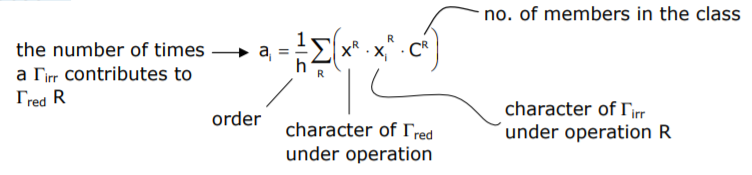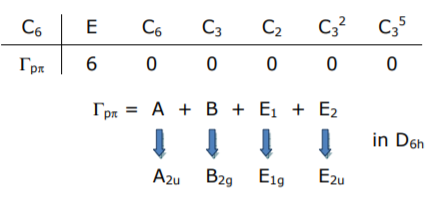# 1.6: LCAO and Hückel Theory 1 (Eigenfunctions)

$$\newcommand{\vecs}{\overset { \rightharpoonup} {\mathbf{#1}} }$$ $$\newcommand{\vecd}{\overset{-\!-\!\rightharpoonup}{\vphantom{a}\smash {#1}}}$$$$\newcommand{\id}{\mathrm{id}}$$ $$\newcommand{\Span}{\mathrm{span}}$$ $$\newcommand{\kernel}{\mathrm{null}\,}$$ $$\newcommand{\range}{\mathrm{range}\,}$$ $$\newcommand{\RealPart}{\mathrm{Re}}$$ $$\newcommand{\ImaginaryPart}{\mathrm{Im}}$$ $$\newcommand{\Argument}{\mathrm{Arg}}$$ $$\newcommand{\norm}{\| #1 \|}$$ $$\newcommand{\inner}{\langle #1, #2 \rangle}$$ $$\newcommand{\Span}{\mathrm{span}}$$ $$\newcommand{\id}{\mathrm{id}}$$ $$\newcommand{\Span}{\mathrm{span}}$$ $$\newcommand{\kernel}{\mathrm{null}\,}$$ $$\newcommand{\range}{\mathrm{range}\,}$$ $$\newcommand{\RealPart}{\mathrm{Re}}$$ $$\newcommand{\ImaginaryPart}{\mathrm{Im}}$$ $$\newcommand{\Argument}{\mathrm{Arg}}$$ $$\newcommand{\norm}{\| #1 \|}$$ $$\newcommand{\inner}{\langle #1, #2 \rangle}$$ $$\newcommand{\Span}{\mathrm{span}}$$$$\newcommand{\AA}{\unicode[.8,0]{x212B}}$$

A common approximation employed in the construction of molecular orbitals (MOs) is the linear combination of atomic orbitals (LCAOs). In the LCAO method, the kth molecular orbital, $$ψ_k$$, is expanded in an atomic orbital basis,

$| \psi_{ k } \rangle = c_{ a } \phi_{ a } + c_{ b } \phi_{ b }+\ldots c_{ i } \phi_{ i } \label{eq1}$

where the $$\phi_{i}$$s are normalized atomic wavefunctions and . Solving Schrödinger’s equation and substituting for $$\psi_{k}$$ yields,

\begin{align*} H \psi_{ k } &= E \psi_{ k } \\[4pt] | H - E | \psi_{ k } \rangle &=0 \end{align*}

Substitute Equation \ref{eq1}

$\left.| H - E | c _{ a } \phi_{ a }+ c _{ b } \phi_{ b }+\ldots+ c _{ i } \phi\right\rangle=0$

Left-multiplying by each $$\phi_{i}$$ yields a set of i linear homogeneous equations,

\begin{align*} \mathrm{c}_{\mathrm{a}}\left\langle\phi_{\mathrm{a}}|\mathrm{H}-\mathrm{E}| \phi_{\mathrm{a}}\right\rangle+\mathrm{c}_{\mathrm{b}}\left\langle\phi_{\mathrm{a}}|\mathrm{H}-\mathrm{E}| \phi_{\mathrm{b}}\right\rangle+\ldots+\mathrm{c}_{i}\left\langle\phi_{\mathrm{a}}|\mathrm{H}-\mathrm{E}| \phi_{i}\right\rangle &=0\\[4pt] c_{a}\left\langle\phi_{b}|H-E| \phi_{a}\right\rangle+c_{b}\left\langle\phi_{b}|H-E| \phi_{b}\right\rangle+\ldots+c_{i}\left\langle\phi_{b}|H-E| \phi_{i}\right\rangle &=0\\[4pt] \vdots\\[4pt] c_{a}\left\langle\phi_{i}|H-E| \phi_{a}\right\rangle+c_{b}\left\langle\phi_{i}|H-E| \phi_{b}\right\rangle+\ldots+c_{i}\langle\phi_i|H-E| \phi_i\rangle&=0 \end{align*}

Solving the secular determinant,

$\begin{array}{ccccc} \mathrm{H}_{\mathrm{aa}}-\mathrm{ES}_{\text {aa }} & \mathrm{H}_{\mathrm{ab}}-\mathrm{ES}_{\text {ab }} & \cdots & \cdots & \mathrm{H}_{\mathrm{ai}}-\mathrm{ES}_{\mathrm{ai}} \\ \mathrm{H}_{\mathrm{ba}}-\mathrm{ES}_{\text {ba }} & \mathrm{H}_{\mathrm{bb}}-\mathrm{ES}_{\mathrm{bb}} & \cdots & \cdots & \mathrm{H}_{\mathrm{bi}}-\mathrm{ES}_{\mathrm{bi}} \\ \vdots & & \ddots & & \vdots \\ \vdots & & & \ddots & \vdots \\ \mathrm{H}_{\mathrm{ia}}-\mathrm{ES}_{\mathrm{ia}} & \mathrm{H}_{\mathrm{ib}}-\mathrm{ES}_{\mathrm{ib}} & \cdots & \cdots & \mathrm{H}_{\mathrm{ii}}-\mathrm{ES}_{\mathrm{ii}} \end{array} \mid=0 \nonumber$

where $$H _{ ij }=\int \phi H \phi d \tau ; \quad S _{ ii }=\int \phi \phi d \tau=1 ; \quad H _{ ij }=\int \phi H \phi_{ j } d \tau ; \quad S _{ ij }=\int \phi \phi_{ j } d \tau$$

In the Hückel approximation,

• $$H _{ iv }=\alpha$$
• $$H _{ ij }=0$$ for $$\phi_{ i }$$ not adjacent to $$\phi_{ j }$$
• $$H _{ ij }=\beta$$ for $$\phi_{ i }$$ not adjacent to $$\phi_{ j }$$
• $$S _{i j}=1$$
• $$S _{ ij }=0$$

The foregoing approximation is the simplest. Different computational methods treat these integrals differently. Extended Hückel Theory (EHT) includes all valence orbitals in the basis (as opposed to the highest energy atomic orbitals), all Sijs are calculated, the Hiis are estimated from spectroscopic data (as opposed to a constant, α) and Hijs are estimated from a simple function of $$S_{ii}$$, $$H_{ii}$$ and $$H_{ij}$$ (zero differential overlap approximation).

The EHT (and other Hückel methods) are termed semi–empirical because they rely on experimental data for quantification of parameters. Other semi-empirical methods include CNDO, MINDO, INDO, etc. in which more care is taken in evaluating Hij (these methods are based on self-consistent field procedures). Still higher level computational methods calculate the pertinent energies from first principles – ab initio and DFT. Here core potentials must be included and high order basis sets are used for the valence orbitals.

## Benzene

As an example of the Hückel method, we will examine the frontier orbitals (i.e. determine eigenfunctions) and their associated orbital energies (i.e. eigenvalues) of benzene. The highest energy atomic orbitals of benzene are the C pπ orbitals. Hence, it is reasonable to begin the analysis by assuming that the frontier MO’s will be composed of LCAO of the C 2pπ orbitals:The matrix representations for this orbital basis in D6h is,

$$E \cdot\left[\begin{array}{l}\phi_{1} \\ \phi_{2} \\ \phi_{3} \\ \phi_{4} \\ \phi_{5} \\ \phi_{6}\end{array}\right]=\left[\begin{array}{llllll}1 & 0 & 0 & 0 & 0 & 0 \\ 0 & 1 & 0 & 0 & 0 & 0 \\ 0 & 0 & 1 & 0 & 0 & 0 \\ 0 & 0 & 0 & 1 & 0 & 0 \\ 0 & 0 & 0 & 0 & 1 & 0 \\ 0 & 0 & 0 & 0 & 0 & 1\end{array}\right]\left[\begin{array}{l}\phi_{1} \\ \phi_{2} \\ \phi_{3} \\ \phi_{4} \\ \phi_{5} \\ \phi_{6}\end{array}\right]=\left[\begin{array}{l}\phi_{1} \\ \phi_{2} \\ \phi_{3} \\ \phi_{4} \\ \phi_{5} \\ \phi_{6}\end{array}\right] \quad x_{\text {trace }}=6$$

$$C _{6} \cdot\left[\begin{array}{l}\phi_{1} \\ \phi_{2} \\ \phi_{3} \\ \phi_{4} \\ \phi_{5} \\ \phi_{6}\end{array}\right]=\left[\begin{array}{llllll}0 & 1 & 0 & 0 & 0 & 0 \\ 0 & 0 & 1 & 0 & 0 & 0 \\ 0 & 0 & 0 & 1 & 0 & 0 \\ 0 & 0 & 0 & 0 & 1 & 0 \\ 0 & 0 & 0 & 0 & 0 & 1 \\ 1 & 0 & 0 & 0 & 0 & 0\end{array}\right]\left[\begin{array}{l}\phi_{1} \\ \phi_{2} \\ \phi_{3} \\ \phi_{4} \\ \phi_{5} \\ \phi_{6}\end{array}\right]=\left[\begin{array}{c}\phi_{2} \\ \phi_{3} \\ \phi_{4} \\ \phi_{5} \\ \phi_{6} \\ \phi_{1}\end{array}\right] \quad x_{\text {trace }}=0$$

$$C _{2}^{\prime} \cdot\left[\begin{array}{c}\phi_{1} \\ \phi_{2} \\ \phi_{3} \\ \phi_{4} \\ \phi_{5} \\ \phi_{6}\end{array}\right]=\left[\begin{array}{rrrrrr}-1 & 0 & 0 & 0 & 0 & 0 \\ 0 & 0 & 0 & 0 & 0 & -1 \\ 0 & 0 & 0 & 0 & -1 & 0 \\ 0 & 0 & 0 & -1 & 0 & 0 \\ 0 & 0 & -1 & 0 & 0 & 0 \\ 0 & -1 & 0 & 0 & 0 & 0\end{array}\right]\left[\begin{array}{c}\phi_{1} \\ \phi_{2} \\ \phi_{3} \\ \phi_{4} \\ \phi_{5} \\ \phi_{6}\end{array}\right]=\left[\begin{array}{c}\bar{\phi}_{1} \\ \bar{\phi}_{6} \\ \bar{\phi}_{5} \\ \bar{\phi}_{4} \\ \bar{\phi}_{3} \\ \bar{\phi}_{2}\end{array}\right] \quad x _{\text {trace }}=-2$$

The only orbitals that contribute to the trace are those that transform into +1 or –1 themselves (i.e. in phase or with opposite phase, respectively). Thus the trace of the remaining characters of the pπ basis may be determined by inspection:

\begin{array}{c|cccccccccccc}
\mathrm{D}_{6 \mathrm{~h}} & \mathrm{E} & 2 \mathrm{C}_{6} & 2 \mathrm{C}_{3} & \mathrm{C}_{2} & 3 \mathrm{C}_{2}^{\prime} & 3 \mathrm{C}_{2}^{\prime \prime} & \mathrm{i} & 2 \mathrm{~S}_{3} & 2 \mathrm{~S}_{6} & \sigma_{\mathrm{h}} & 3 \sigma_{\mathrm{v}} & 3 \sigma_{\mathrm{d}} \\
\hline \Gamma_{\mathrm{p} \pi} & 6 & 0 & 0 & 0 & -2 & 0 & 0 & 0 & 0 & -6 & 2 & 0
\end{array}

The Γ representation is a reducible basis that must be decomposed into irreducible representations.

Decomposition of reducible representations may be accomplished with the following relation:Returning to the above example,

$a_{A_{19}}=\frac{1}{24}[6 \cdot 1 \cdot 1+0 \cdot 0 \cdot 0+(-2)(1)(3)+0+0+0+0+(-6)(1)(1)+2 \cdot 1 \cdot 3+0]=0 \nonumber$

thus A1g does not contribute to Γ

How about $$a_{A_{2 u}}$$?

$a_{A_{2 u}}=\frac{1}{24}[6 \cdot 1 \cdot 1+0 \cdot 0 \cdot 0+(-2)(-1)(3)+0+0+0+0+(-6)(1)(-1)+2 \cdot 1 \cdot 3+0]=1 \nonumber$

Continuing the procedure, one finds,

$\Gamma_{ p \pi}= A _{2 u }+ B _{29}+ E _{19}+ E _{2 u }$

these are the symmetries of the MO’s formed by the LCAO of pπ orbitals in benzene.

With symmetries established, LCAOs may be constructed by “projecting out” the appropriate linear combination. A projection operator, P(i) , allows the linear combination of the ith irreducible representation to be determined,A drawback of projecting out of the D6h point group is the large number of operators. The problem can be simplified by dropping to the pure rotational subgroup, C6. In this point group, the full extent of mixing among $$\phi_{1}$$ through $$\phi_{6}$$ is maintained; however the inversion center, and hence u and g symmetry labels are lost. Thus in the final analysis, the Γis in C6 will have to be correlated to those in D6h. Reformulating in C6,The projection of the SALC that from $$\phi_{1}$$ transforms as A is,Continuing,

• $$P ^{( B )} \phi_{1}=\phi_{1}-\phi_{2}+\phi_{3}-\phi_{4}+\phi_{5}-\phi_{6}$$
• $$P ^{\left( E _{1 a}\right)} \phi_{1}=\phi_{1}+\varepsilon \phi_{2}-\varepsilon^{*} \phi_{3}-\phi_{4}-\varepsilon \phi_{5}+\varepsilon^{*} \phi_{6}$$
• $$P ^{\left( E _{16}\right)} \phi_{1}=\phi_{1}+\varepsilon^{*} \phi_{2}-\varepsilon \phi_{3}-\phi_{4}-\varepsilon^{*} \phi_{5}+\varepsilon \phi_{6}$$
• $$P ^{\left( E _{22}\right)} \phi_{1}=\phi_{1}-\varepsilon^{*} \phi_{2}-\varepsilon \phi_{3}+\phi_{4}-\varepsilon^{*} \phi_{5}-\varepsilon \phi_{6}$$
• $$P ^{\left( E _{26}\right)} \phi_{1}=\phi_{1}-\varepsilon \phi_{2}-\varepsilon^{*} \phi_{3}+\phi_{4}-\varepsilon \phi_{5}-\varepsilon^{*} \phi_{6}$$

The projections contain imaginary components; the real component of the linear combination may be realized by taking ± linear combinations:

For $$\psi\left( E _{1 a }\right)$$ SALC’s:

$$\psi_{3}^{\prime}\left(E_{1 a}\right)+\psi_{4}^{\prime}\left(E_{1 b}\right)=2 \phi_{1}+\left(\varepsilon+\varepsilon^{*}\right) \phi_{2}-\left(\varepsilon+\varepsilon^{*}\right) \phi_{3}-2 \phi_{4}-\left(\varepsilon+\varepsilon^{*}\right) \phi_{5}+\left(\varepsilon+\varepsilon^{*}\right) \phi_{6}$$
$$\psi_{3}^{\prime}\left(E_{1 a}\right)-\psi_{4}^{\prime}\left(E_{1 b}\right)=\left(\varepsilon-\varepsilon^{*}\right) \phi_{2}+\left(\varepsilon-\varepsilon^{*}\right) \phi_{3}+\left(\varepsilon^{*}-\varepsilon\right) \phi_{5}+\left(\varepsilon^{*}-\varepsilon\right) \phi_{6}$$

where in the C6 point group,

$$\varepsilon=\exp \left(\frac{2 \pi}{6}\right) i =\cos \frac{2 \pi}{6}- i \sin \frac{2 \pi}{6}$$
$$\therefore \varepsilon+\varepsilon^{*}=\cos \frac{2 \pi}{6}- i \sin \frac{2 \pi}{6}+\cos \frac{2 \pi}{6}+ i \sin \frac{2 \pi}{6}=2 \cos \frac{2 \pi}{6}=1$$
$$\varepsilon^{*}-\varepsilon=-\cos \frac{2 \pi}{6}+ i \sin \frac{2 \pi}{6}-\cos \frac{2 \pi}{6}+ i \sin \frac{2 \pi}{6}=2 i \sin \frac{2 \pi}{6}= i \sqrt{3}$$
$$\varepsilon-\varepsilon^{*}=\cos \frac{2 \pi}{6}- i \sin \frac{2 \pi}{6}-\left(\cos \frac{2 \pi}{6}+ i \sin \frac{2 \pi}{6}\right)=-2 i \sin \frac{2 \pi}{6}=- i \sqrt{3}$$

∴ the E1a LCAO’s reduce to (again ignoring the constant prefactor),

$$\psi_{3}\left( E _{1}\right)=\psi_{3}^{\prime}\left( E _{1 a }\right)+\psi_{4}^{\prime}\left( E _{1 b }\right)=2 \phi_{1}+\phi_{2}-\phi_{3}-2 \phi_{4}-\phi_{5}+\phi_{6}$$
$$\psi_{4}\left( E _{1}\right)=\psi_{3}^{\prime}\left( E _{1 a }\right)-\psi_{4}^{\prime}\left( E _{1 b }\right)=\phi_{2}+\phi_{3}-\phi_{5}-\phi_{6}$$

Similarly for the ψ5(E2) and ψ6(E2) LCAO’s… normalizing the SALC’s

$$\begin{array}{ll}\psi_{1}( A )=\frac{1}{\sqrt{6}}\left(\phi_{1}+\phi_{2}+\phi_{3}+\phi_{4}+\phi_{5}+\phi_{6}\right) & \psi_{2}( B )=\frac{1}{\sqrt{6}}\left(\phi_{1}-\phi_{2}+\phi_{3}-\phi_{4}+\phi_{5}+\phi_{6}\right) \\ \psi_{3}\left( E _{1}\right)=\frac{1}{\sqrt{12}}\left(2 \phi_{1}+\phi_{2}-\phi_{3}-2 \phi_{4}-\phi_{5}+\phi_{6}\right) & \psi_{4}\left( E _{1}\right)=\frac{1}{2}\left(\phi_{2}+\phi_{3}-\phi_{5}-\phi_{6}\right) \\ \psi_{5}\left( E _{2}\right)=\frac{1}{\sqrt{12}}\left(2 \phi_{1}-\phi_{2}-\phi_{3}+2 \phi_{4}-\phi_{5}-\phi_{6}\right) & \psi_{6}\left( E _{2}\right)=\frac{1}{2}\left(\phi_{2}-\phi_{3}+\phi_{5}-\phi_{6}\right)\end{array}$$

The pictorial representation of the SALC’s are,This page titled 1.6: LCAO and Hückel Theory 1 (Eigenfunctions) is shared under a CC BY-NC-SA 4.0 license and was authored, remixed, and/or curated by Daniel Nocera via source content that was edited to the style and standards of the LibreTexts platform; a detailed edit history is available upon request.